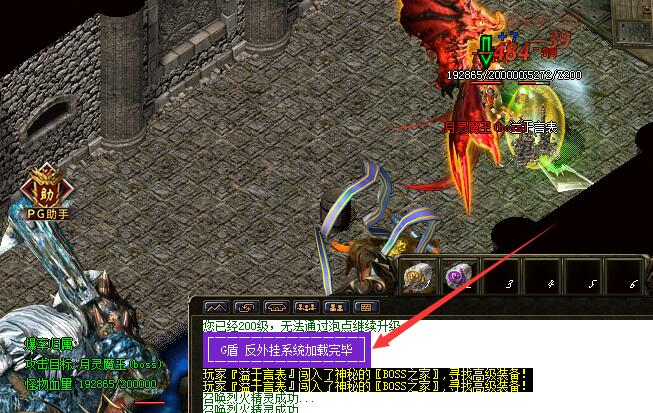# 秒杀辅助G盾登陆器挂机定制版脚本范本function 回收() --回收子程序
点击("@回收",0)
等待(500)
点击坐标(271,182)
等待(500)
点击坐标(256,214)
等待(500)
物品存仓()
等待(500)
按键("esc")
点击("@回收",0)
等待(500)
点击("353,341",0)
等待(500)
点击("285,243",0)
等待(500)
点击("520,244",0)
等待(500)
math.randomseed(os.time())
置标记(123,math.random(2,9))
end

if (获取地图名()=="风云神墓") then --此地图是打怪地图,如发现地图名字错误请修改
设置包裹格数(120)
if 获取窗口("NPC对话框")==1 then
按键("esc")
end
if 获取窗口("对话框")==1 then
按键("enter")
end
if 获取窗口("包裹")==1 then
按键("f9")
end
设置挂机点("30,60|57,78|73,60|70,29")
丢弃物品()
if (角色("死亡")==1) then
--关闭提示框(0)--ESP可使用,关闭屏蔽提示框的选择
等待(300)
小退()
--关闭提示框(1)--ESP可使用,继续开启屏蔽提示框的选择
end
if 取标记(123)==0 then
math.randomseed(os.time())
置标记(123,math.random(2,9))
end
if (取背包空位()<取标记(123)) then --检测包裹内空位少于10格执行回收子程序
回收()
else
开始打怪()
end
end

if (获取地图名()=="盟重省") then   --此地图进图,如发现地图名字错误请修改
血量=角色("当前HP")
最大血量=角色("最大HP")
血量百分比=(血量/最大血量)*100
if  检测复活()==1 then --判断可不删除
按键("呵呵")
return
end
if 获取窗口("包裹")==1 then
按键("f9")
end
if 获取窗口("NPC对话框")==1 then
按键("esc")
end
if 获取窗口("对话框")==1 then
按键("enter")
end
if 血量百分比<90 then --血量低于90%不进图
按键("呵呵")
return
end
if 寻路(338,329,2)==1 then  --寻路到此处开始对话
对话坐标(340,324)  --对话的NPC坐标
等待(800)
if 获取窗口("NPC对话框")==1 then
点击("@9008",0)
等待(500)
if 获取窗口("对话框")==1 then
按键("enter")
等待(500)
end
end
end --此处为对话NPC结束
end